Spitzer Documentation & Tools
IRS Instrument Handbook

## 2.10            Sensitivity

The median 1-sigma continuum sensitivity for the IRS low-resolution modules is about 0.06 mJy from 6 to 15 microns and 0.4 mJy from 14 to 38 microns in 512 seconds of integration with low background.  The median 1-sigma line sensitivity estimates for the short- and long-wavelength high-resolution modules are about 2.7x10-19 W/m2 and 8.5x10-19 W/m2, respectively, for 512 seconds of integration with low background (see Figure 2.14 through Figure 2.18).

### 2.10.1         Equations for Calculating Sensitivity and Signal-To-Noise for Point Sources

This section illustrates the procedure by which the expected instrumental sensitivity and signal-to-noise ratios were calculated.

The background photocurrent from sky and telescope is given by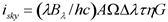Equation 2.1

where h is the Planck constant and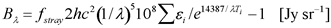Equation 2.2

where 2hc2 = 1.19x10-4  W micron2, all lengths are in microns, fstray is the ratio of total sky throughput to that of an ideal f/12 telescope, and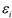are sky emissivities at temperatures Ti.  We also define the following: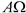= the telescope throughput in cm2 sr.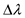=the spectral bandpass falling on one pixel (=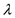/R) in cm.  Note: while there are 2 pixels per resolution element, the slit width is also two pixels.  Hence,is proportional to the slit width if the spectral plate scale is unchanged.

Ti = effective blackbody temperature of sky + telescope.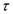= the cold optical throughput for extended sources (i.e., omitting slit losses).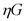= the detector responsivity (electrons/photon) [=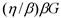].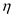=quantum efficiency of the detector at the desired wavelength.

βG = the dispersion-gain product.

In the faint source limit, the total noise in electrons/pixel is the sum of the photocurrent shot noise (isky), read noise, and dark current shot noise.  It is given by: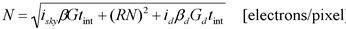Equation 2.3

where id is the dark current contribution, RN is the read noise in electrons, tint is the integration time in seconds, and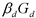is the dispersion-gain product for the dark observations.

The relationship between photocurrent/pixel and the incident flux density of a source in mJy is given by: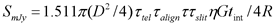[electrons/pixel/mJy] Equation 2.4

where 1 mJy = 10-26 erg s-1 cm-2 Hz-1, D is the telescope aperture (in cm2),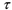is?the slit throughput of a point source with angular diameter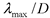(without considering losses), and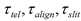are throughputs accounting for losses in the telescope, alignment effects, and slit, respectively.  The second factor of 4 in Equation 2.4 comes from dividing the point source flux over 2 pixels per spectral resolution element and 2 pixels spatial extent. Again assuming the faint source limit, the 1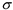staring point source continuum sensitivity (PSSC) in mJy at the full resolution of the spectrograph is: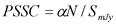[mJy] Equation 2.5

where the factor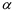accounts for the variation in PSF with wavelength and details of the point source extraction.  Its value lies between 0.8 and 1.2.  The 1staring point source continuum sensitivity (in mJy) smoothed at the required resolution of the spectrograph (Rreq = 50) is referred to as the PSSCS, and is given by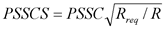Equation 2.6

The spectra are smoothed from R to Rreq and the S/N is assumed to increase as the square root of the number of pixels averaged.  Suitable sub-pixel smoothing is assumed.

The 1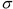staring point source sensitivity (in W m-2) for unresolved lines is then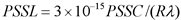Equation 2.7

where the factor 3x10-15 = c (micron/s) (10-29 W m-2 Hz-1/mJy), PSSC is in units of mJy, and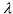is in microns.